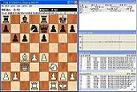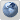WBEC-Ridderkerk forum
Would you like to react to this message? Create an account in a few clicks or log in to continue.

# WBEC Ridderkerk, Premier B 2nd test result..##WBEC Ridderkerk, Premier B 2nd test result..`Test at Quad9550 at 40/5, x64 and 2 cores/engine if supported by the engine! ponder=on DONATION_WBEC, 2009.02.17 - 2009.02.18                                Score     T K C Z F G P R M T S S C M---------------------------------------------------------------------- 1: Thinker 5.4b-x64-2cpu      9.0 / 13   X = 1 1 = 1 1 1 = 0 = 1 0 1 2: Ktulu 9.0                  9.0 / 13   = X 0 0 = 1 0 1 1 1 1 1 1 1 3: Colossus 2008b             8.5 / 13   0 1 X = 1 = 0 = 1 1 = = 1 1 4: Zappa Mexico II-x64-2cpu   8.5 / 13   0 1 = X = = = 1 = 1 = = 1 1 5: Fruit 2.3.5m-x64           8.5 / 13   = = 0 = X 1 0 = 1 = 1 1 1 1 6: Gandalf 6.01               8.0 / 13   0 0 = = 0 X 1 1 = 1 = 1 1 1 7: Pro Deo 1.6                7.5 / 13   0 1 1 = 1 0 X 0 0 1 1 0 1 1 8: Ruffian 2.1.0              6.5 / 13   0 0 = 0 = 0 1 X 1 = = = 1 1 9: Movei 0.08.438             5.5 / 13   = 0 0 = 0 = 1 0 X 0 1 1 = =10: The Baron 3.3-x64-2cpu     5.5 / 13   1 0 0 0 = 0 0 = 1 X = 1 = =11: Spike 1.3x4                5.5 / 13   = 0 = = 0 = 0 = 0 = X = 1 112: SlowChess blitz WV2.1      4.0 / 13   0 0 = = 0 0 1 = 0 0 = X 0 113: Chiron 0.87                4.0 / 13   1 0 0 0 0 0 0 0 = = 0 1 X 114: Maestro 3.0                1.0 / 13   0 0 0 0 0 0 0 0 = = 0 0 0 X----------------------------------------------------------------------91 games: +34 =29 -28`

Problems found:

LGpgnver:
File: 16_P_BT2.pgn
Level of warnings: 0
=============================================================================
0 warnings/errors found

Best wishes,
Leo.

Games of this testLeo Dijksman

Number of posts : 454
Age : 70
Location : Stavenisse
Registration date : 2008-05-17Permissions in this forum:
You cannot reply to topics in this forum